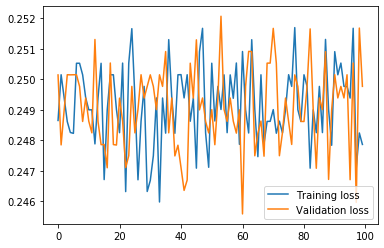# LSTM loss keeps fluctuating

Hello I am a beginner and I am using pytorch to implement LSTM model for classification. when running the model the loss values keep changing (Increase and decrease randomly). shown by the following graph. When I try to implement it by other frameworks like Keras using the same data the results are correct and the loss decreases with time.I am using MSELoss function and following is my code for the LSTM class

``````class LSTM(nn.Module):
def __init__(self, input_dim=272, hidden_layer_size=64, output_size=1,batch=64,n_layer=1):
super().__init__()

self.hidden_layer_size = hidden_layer_size
self.lstm = nn.LSTM(input_dim, hidden_layer_size,batch_first=True)
self.linear1 = nn.Linear(hidden_layer_size, 32)
self.relu = nn.ReLU()
self.linear2 = nn.Linear(32, output_size)
self.hidden_cell = (torch.randn(n_layer,batch,self.hidden_layer_size).cuda(), torch.randn(n_layer,batch,self.hidden_layer_size).cuda())
self.sigmoid = nn.Sigmoid()

def forward(self, input_seq):
lstm_out, self.hidden_cell = self.lstm(input_seq, self.hidden_cell)
#print(lstm_out[:,-1,:].shape)
linear1_out = self.linear1(lstm_out[:,-1,:])
relu=self.relu(linear1_out)
linear2_out = self.linear2(relu)
prediction=self.sigmoid(linear2_out)
return prediction

def backward(self,g0):
raise RuntimeError("Some Error in Backward")
return g0.clone()
``````
• Why are you using MSELoss if it is a classification task (you have used a sigmoid function at the end of network squishing the value between 0 and 1)? Was this intentional? Did you use the same methodology in Keras?
• Did you set the same learning rates in both the models? Can you also print out the Keras code? Both the codes could then be crosschecked.